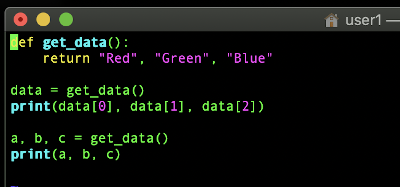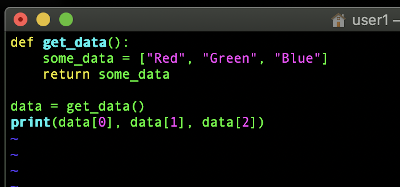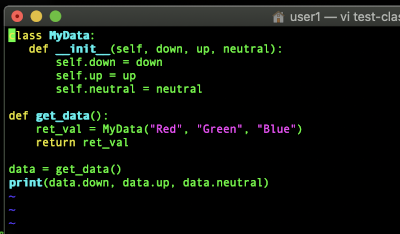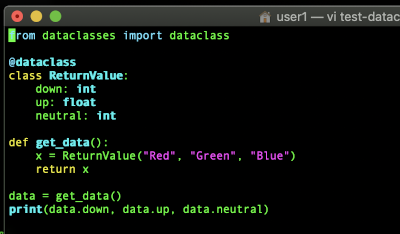# Return Multiple Values Python

If you are reading this then you are probably wondering how to return multiple values in Python. It is really easy and there are multiple different ways to achieve this. We will cover the different methods available. We are going to have a video as well as several code examples if you keep scrolling past the video. If you want a quick answer just return a tuple like this: “return a, b, c”.

• using a tuple
• using a named tuple
• using a list
• using a dict
• using a class/object
• using a dataclass ( Python 3.7 )

## Using a Tuple:

The most common way people return multiple values in Python is by using a tuple. This is easy and straight forward. It is even more simple when you don’t even explicitly define a variable for your tuple. Notice that the value returned can be assigned to a single variable holding your tuple or it can be automatically broken up into separate variables as shown below.

``````
def get_data():
return "Red", "Green", "Blue"

data = get_data()
print(data, data, data)

a, b, c = get_data()
print(a, b, c)
``````## Using a Named Tuple:

Using a named tuple is a bit better than using a normal tuple even though it may seem unnecessary in many cases. It makes accessing values a lot easier. It results in code that is easier for others to understand and nicer to work with. This is considered by some to be the preferred solution.

``````
from collections import namedtuple

def get_data():
ret_val = namedtuple("color", "down up neutral")
return ret_val("Red", "Green", "Blue")

data = get_data()
print(data.down, data.up, data.neutral)
``````## Using a List

This is another option. It is similar to using a regular tuple except that it is mutable. The value returned can be changed. That sounds like what you need then you should use this instead of a tuple. Here is an example of how we would return multiple values in Python using a list.

``````
def get_data():
some_data = ["Red", "Green", "Blue"]
return some_data

data = get_data()
print(data, data, data)
``````## Using a Dict

Using a dict is yet another option. This is what you will want if you want to have named values and you want those values to be mutable. If you will need to edit the returned value but want to use keys instead of index numbers then you should use a dict.

``````
def get_data():
some_data = {'down':'Red', 'up':'Green', 'neutral':'Blue'}
return some_data

data = get_data()
print(data['down'], data['up'], data['neutral'])
``````## Using a Class/Object to Return Multiple Values Python

Using a class is another step up in sophistication. This is a little bit more elaborate. You normally wouldn’t use this just so you can return multiple values. You would normally do this if you want something with a specific structure and potentially member functions. If you wouldn’t be using a class anyway, then don’t use one just so that you can return multiple values. All of the other options we’ve discussed are more simple. There are plenty of use cases for this though

Here we define a class first. Then we define a separate function. Inside the function we create an instance of a our class initialized with the values that we want to return. Then we return them. From there calling the function and using the values is pretty straight forward. The values can be accessed by name.

``````
class MyData:
def __init__(self, down, up, neutral):
self.down = down
self.up = up
self.neutral = neutral

def get_data():
ret_val = MyData("Red", "Green", "Blue")
return ret_val

data = get_data()
print(data.down, data.up, data.neutral)

``````## Using a Dataclass to Return Multiple Values Python

You can use Data Classes to return multiple values as well. This option is available in Python 3.7. Here is our example below.

``````
from dataclasses import dataclass

@dataclass
class ReturnValue:
down: int
up: float
neutral: int

def get_data():
x = ReturnValue("Red", "Green", "Blue")
return x

data = get_data()
print(data.down, data.up, data.neutral)
``````

Here is a screenshot vi displaying my sample program from a terminal:## Return Multiple Values Into Multiple Variables

Besides just returning something that contains multiple values you can actually have these values assigned to multiple variables. See the example below. This can be really handy.

``````
def test2():
return 'tst', 123, 'data'

a, b, c = test2()
``````